# Bricks

Brick has volume 2.4 dm3. How many bricks can drive a truck with a capacity of 15 ton? The density of brick is 2 g/cm3.

n =  3125

### Step-by-step explanation:Did you find an error or inaccuracy? Feel free to write us. Thank you!Tips to related online calculators
Do you know the volume and unit volume, and want to convert volume units?
Tip: Our Density units converter will help you with the conversion of density units.
Do you want to convert mass units?
Do you want to round the number?

## Related math problems and questions:

• BricksOpenings in perforated bricks occupy 10% and brick has dimensions 30 cm, 15 cm and 7.5 cm. Calculate a) the weight of a perforated bricks, if you know that the density of the full brick material is p = 1800 kg/m3 (1.8 kg/dm3) b) the number of perforated
• Waste container eco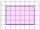The waste box has the shape of a block with dimensions of 1.8 m, 1.5 m, 1.2 m. 0.25 m3 of waste is added to it every day. How many days will it be filled?
• The granite ballWhat is the weight of a granite ball with a radius of 60 cm, if 1 m3 of granite weighs 2.7 tons?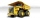The truck with a load capacity of 7 tons has a storage area of 4.5 m and 2.1 m. The weight of 1 m3 of wet sand is 2,000 kg. How high can we load the sand so that the load capacity of the car is not exceeded?
• Cubes into cuboidHow many 12 centimeter cubes fit into the block (cuboid) with 6dm, 8.4dm, and 4.8?
• BricksHow many bricks are needed for a wall 14.5 m long, 2 m high and 45 cm thick, if 285 bricks are needed for one m ^ 3 wall?
• Living roomHow many people can live in a room with dimensions: a = 4m b = 5m c = 2.5m if one person needs 15m cubic space (i. E. Air . .. )?
• Cargo spaceThe cargo space of the truck has the dimensions a = 4.2 m, b = 1.9 m, c = 8.1 dm. Calculate its volume.
• AquariumCan 30 liters of water fit in a cuboid aquarium with dimensions a = 3dm b = 6dm c = 5dm?
• Oak cuboidOak timber is rectangular shaped with dimensions of 2m, 30 cm and 15 cm. It weight is 70 kg. Calculate the weight 1 dm³ of timber.
• Brick wallWhat is the weight of a solid brick wall that is 30 cm wide, 4 m long and 2 m high? The density of the brick is 1500 kg per cubic meter.
• Granite cube statue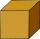What is the weight of a granite cube with an edge length of 75 cm, if 1 m3 of granite has a weight of 2.7 tons?
• Bricks II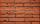3/4 bricks weighs 6 kg and 2/3 bricks. How weighs one whole brick?
• Bricks wallThere are 5000 bricks. How high wall thickness of 20 cm around the area which has dimensions 20 m and 15 m can use these bricks to build? Brick dimensions are 30 cm, 20 cm and 10 cm.
• PercentagesCalculate the percentages to two decimal places: a / 15 min in 4 hours B / 35 cm2 of 12.5 dm2 c / 2dm2 of 400 cm2 D / 0.2 liters from 2.2 liters
• The wall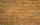We have to build a cuboid wall with dimensions base 30 cm and 45 cm and height 3.25 meters. Calculate how many we need bricks if we spend 400 pieces of bricks to 1 m3 of wall?
• Two cuboidsFind the volume of the cuboidal box whose one edge is: a) 1.4m and b) 2.1 dm.